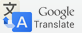15-02-2019

# This is a guide to help select the anti-vibration rubber Pads.

1. Load per mount. When the load is evenly distributed, divide the total load of the equipment (lbs) to be isolated by the number of mounts.
2. Pressure per mount. Divide the load per mount (lbs) by the equipment mount/foot area (square in).

Load per mount (lbs) = total load (lbs) ÷ number of mounts

Pressure per mount (psi) = Load per mount (lbs) ÷ mount/foot area (square in)

Step 02: Frequency

1. When the vibration frequency is not known, calculations of the disturbing frequency (Hz) can be done based on the operating speed (RPM).

Disturbing frequency (Hz) = operating speed (RPM) ÷ 60

1. Calculate the natural frequency (Hz) that the system needs for 80% isolation.

Natural frequency (Hz) = Disturbing frequency (Hz) ÷ 2.45

Step 03: Deflection

1. Required static deflection (in) is calculated based on the natural frequency (Hz) that the system needs for 80% isolation

Required static deflection (in) = 9.8 ÷ (natural frequency (Hz))^2

1. Required spring rate (lbs/in) is calculated by dividing the load per mount (lbs) by the required static deflection (in)

Required spring rate (lbs/in) = load per mount (lbs) ÷ required static deflection (in)

Step 04: Select an anti-vibration Pad